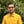Trusted answers to developer questions
Trusted Answers to Developer Questions

Related Tags

d
math
exp2
communitycreator

# What is exp2() in D?Harris Amjad

Grokking Modern System Design Interview for Engineers & Managers

Ace your System Design Interview and take your career to the next level. Learn to handle the design of applications like Netflix, Quora, Facebook, Uber, and many more in a 45-min interview. Learn the RESHADED framework for architecting web-scale applications by determining requirements, constraints, and assumptions before diving into a step-by-step design process.

The exp2() function in D returns $2$ raised to the power of a specific number.

The mathematical representation of the exp2() function is shown below.

Mathematical representation of the exp2() function

Note: We need to import std.math in our code in order to use the exp2() function. We can import it like this:
import std.math

### Syntax

// The number should be floating, double, or real.
exp2(number)


### Parameters

This function requires a number as a parameter.

### Return value

The exp2() function returns $2$ raised to the power of the specific number sent as a parameter.

Note:

• If the parameter value is positive infinity, then exp2() returns positive infinity.
• If the parameter value is negative infinity, exp2() returns 0.
• If the parameter value is NaN, exp2() returns NaN.
• If the parameter value is -NaN, exp2() returns -NaN.

### Code

The code below shows how to use the exp2() function in D.

import core.stdc.stdio;import std.stdio;//Header required for the functionimport std.math;int main(){    //Integer    writeln ("The value of exp2(10.0) : ",exp2(10.0));    //Positive double value    writeln ("The value of exp2(2.56) : ",exp2(2.56));    //Negative double value    writeln ("The value of exp2(-2.56) : ",exp2(-2.56));    //Zero    writeln ("The value of exp2(0.0) : ",exp2(0.0));    //Exceptional outputs    writeln ("The value of exp2(real.infinity) : ",exp2(real.infinity));    writeln ("The value of exp2(-real.infinity) : ",exp2(-real.infinity));    writeln ("The value of exp2(real.nan) : ",exp2(real.nan));    writeln ("The value of exp2(-real.nan) : ",exp2(-real.nan));    return 0;}
Using exp2()

### Explanation

• Line 4: We add the std.math header required for the exp2() function.

• Line 9: We calculate the exponent with base 2 of the integer value using exp2().

• Line 12: We calculate the exponent with base 2 of the positive double value using exp2().

• Line 15: We calculate the exponent with base 2 of the negative double value using exp2().

• Line 18: We calculate the exponent with base 2 of the zero using exp2().

• Line 20 onwards: We calculate the exponent with base 2 of exceptional numbers using exp2().

RELATED TAGS

d
math
exp2
communitycreator

CONTRIBUTORHarris Amjad

Grokking Modern System Design Interview for Engineers & Managers

Ace your System Design Interview and take your career to the next level. Learn to handle the design of applications like Netflix, Quora, Facebook, Uber, and many more in a 45-min interview. Learn the RESHADED framework for architecting web-scale applications by determining requirements, constraints, and assumptions before diving into a step-by-step design process.

Keep Exploring

Learn in-demand tech skills in half the time